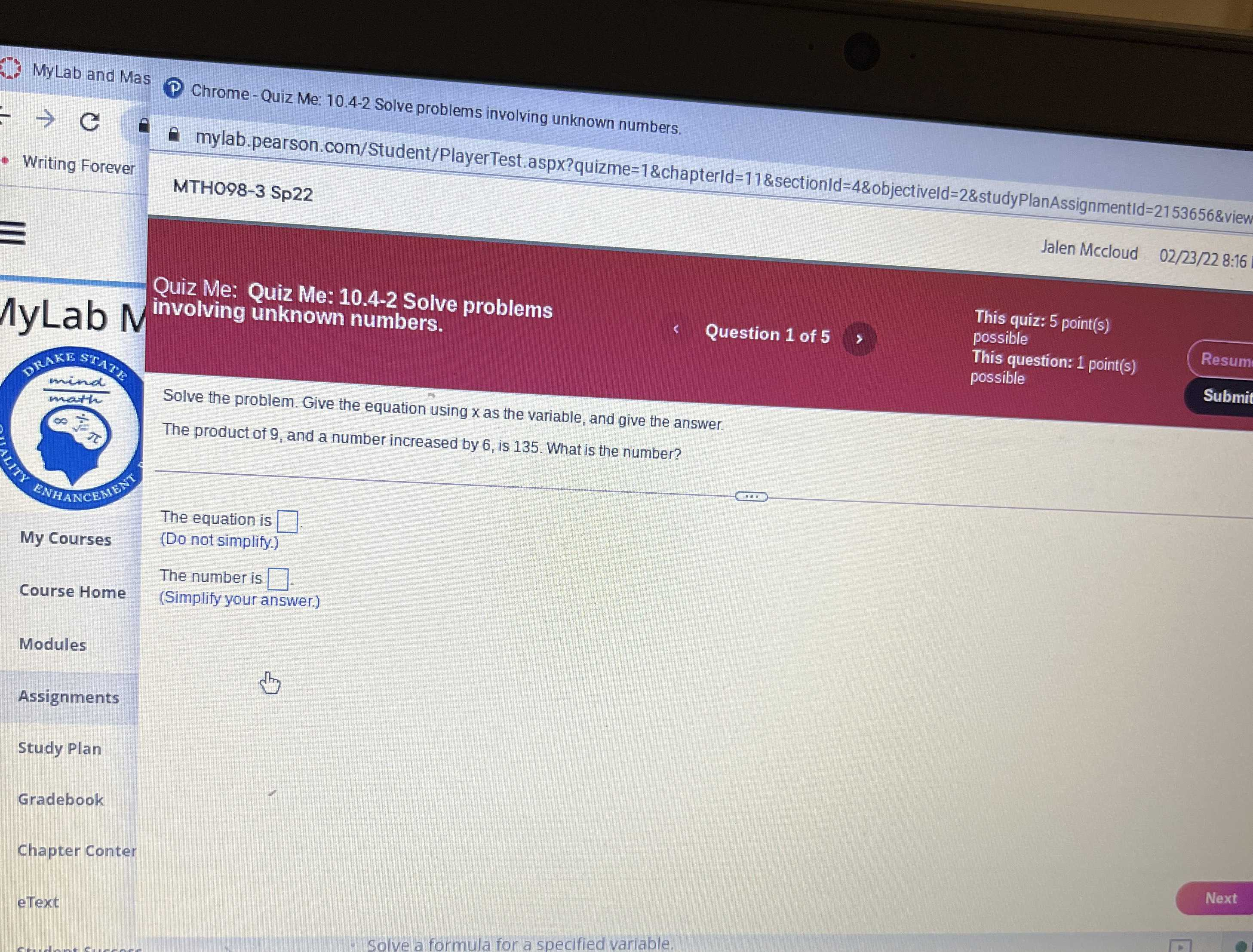### Still have math questions?

Algebra
QuestionSolve the problem. Give the equation using $$x$$ as the variable, and give the answe The product of $$9$$ , and a number increased by $$6$$ , is $$135$$ . What is the number?

The equation is $$\square$$ . (Do not simplify.) The number is $$\square$$ . (Simplify your answer.)

$$9\times (x+ 6)= 135\\x= 9$$